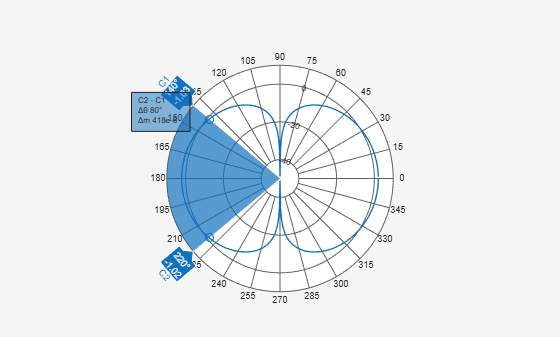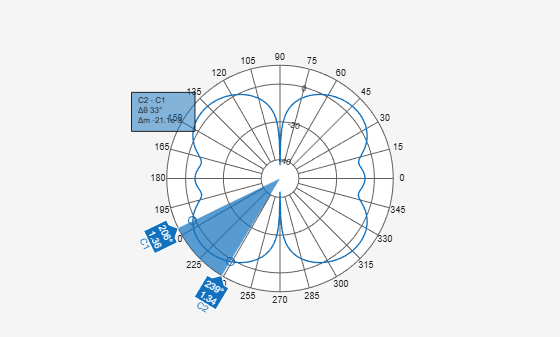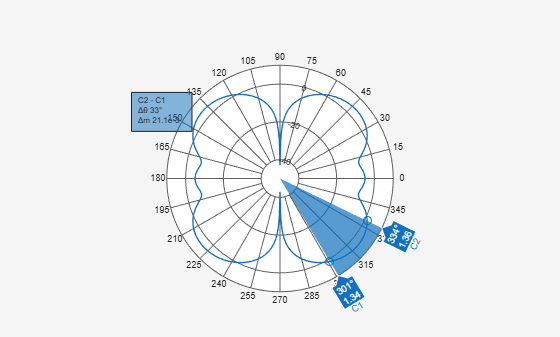beamwidth

Beamwidth of antenna

Description

example

beamwidth(antenna,frequency,azimuth,elevation) plots the beamwidth of the input antenna at a specified frequency. The beamwidth is the angular separation at which the magnitude of the directivity pattern decreases by a certain value from the peak of the main beam. The directivity decreases in the direction specified by azimuth and elevation angles of the antenna.

Note

• beamwidth plots only one beamwidth for symmetrical patterns.

• beamwidth might not interpret the data well for partial angle data.

bw = beamwidth(antenna,frequency,azimuth,elevation,dBdown) returns the beamwidth of the antenna at a specified dBdown value from the peak of the radiation pattern main beam.

example

[bw,angles] = beamwidth(____) returns the beamwidth and angles (points in a plane) using any input arguments from previous syntaxes.

Examples

collapse all

Plot the beamwidth for a dipole antenna at azimuth=0 and elevation=1:1:360 (x-z plane)

d  = dipole;
beamwidth(d,70e6,0,1:1:360);Calculate the beamwidth of a helix antenna and the angles of the beamwidth. The antenna has an azimuth angle of 1:1:360 degrees, an elevation angle of 0 degrees on the X-Y plane, and a dB down value of 5 dB.

hx = helix;
[bw,angles] = beamwidth(hx,2e9,1:1:360,0,5)
bw = 144
angles = 1×2

143   287

Calculate beamwidth and angle of a fractalGasket.

[bw,ang] = beamwidth(fg,1.3e9,0,0:1:360) % bw is a 2-by-1 vector.
bw = 2×1

24.0000
24.0000

ang = 2×2

348    12
168   192

Plot beamwidth.

beamwidth(fg,1.3e9,0,0:1:360)Plot Second Beamwidth Solution

Get the polarpattern handle.

P = polarpattern('gco');

Hide the beamwidth span and remove the cursor C1 and C2. All the cursors can also be removed using the function removeCursors.

showSpan(P,0);
removeCursors(P,1);
removeCursors(P,2);

Add the cursors at other side of the plot and second beamwidth solution is displayed.

showSpan(P,1);Input Arguments

collapse all

Antenna object, specified as a scalar.

Frequency to calculate beamwidth, specified as a scalar in Hz.

Example: 70e6

Data Types: double

Azimuth angle of the antenna, specified as a scalar or vector in degrees. If the elevation angle is specified as a vector, then the azimuth angle must be a scalar.

Example: 3

Data Types: double

Elevation angle of the antenna, specified as a scalar or vector in degrees. If the azimuth angle is specified as a vector, then the elevation angle must be a scalar.

Example: 1:1:360

Data Types: double

Power point from peak of main beam of antenna, specified as a scalar in dB.

Example: 5

Data Types: double

Output Arguments

collapse all

Beamwidth of antenna, returned as a scalar in degrees or a 2-by-1 vector with each element unit in degrees.

Points on plane used to measure beamwidth, returned as a vector with each element unit in degrees.